Courses

# Numerical Methods Civil Engineering (CE) Notes | EduRev

## Civil Engineering (CE) : Numerical Methods Civil Engineering (CE) Notes | EduRev

The document Numerical Methods Civil Engineering (CE) Notes | EduRev is a part of the Civil Engineering (CE) Course Topic wise GATE Past Year Papers for Civil Engineering.
All you need of Civil Engineering (CE) at this link: Civil Engineering (CE)

Question 1: The value of the function f(x) is given at n distinct values of x and its value is to be interpolated at the point x*, using all the n points. The estimate is obtained first by the Lagrange polynomial, denoted by IL and then by the Newton polynomial, denoted by IN. Which one of the following statements is correct?
(a) IL is always greater than IN

(b) No definite relation exists between IL and IN
(c) IL and IN are always equal
(d) IL is always less than IN        [2019 : 1 Mark, Set-ll]

Answer: (c)

Question 2: The quadratic equation 2x2 - 3x + 3 = 0 is to be solved numerically starting with an initial guess as x0 = 2. The new estimate of x after the first iteration using Newton-Raphson method is ______.    [2018 : 1 Mark, Set-II]
Solution:
Given
f(x) = 2x2 - 3x + 3, x0 = 2
f'(x) = 4x - 3
By Newton-Rapshon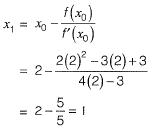Question 3: Consider the equation du/dt = 3t2 + 1 with u = 0 at t = 0. This is numerically solved by using the forward Euler method with a step size. Δt = 2. The absolute error in the solution in the end of the first time step is _____________.    [2017 : 2 Marks, Set-I]
Solution:  du/dt = 3t2 + 1
f(u , f) = 3t2 + 1
u0 = 0
t0 = 0
Δt = 2
By Euler’s method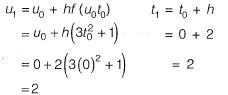After first iteration u = 2 when t = 2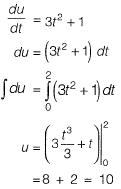Absolute error = Exact value - approx value
= 10 -2
= 8

Question 4: Newton-Raphson method is to be used to find foot of equation 3x - ex + sinx = 0. If the initial trail value of the roots is taken as 0.333, the next approximation for the root would be __________.    [2016 : 1 Mark, Set-I]
Solution: According to Newton-Raphson Method: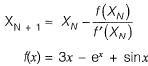or, f' (x) = 3 - ex + cosx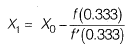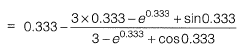∴ X1 = 0.36

Question 5: For step-size, Δx = 0.4, the value of following integral using Simpson’s 1/3 rule is _________.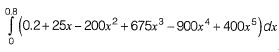[2015 : 2 Marks, Set-II]
Solution: a = 0,b = 0.8, Δx = 0.4
f{x) = 0.2 + 25x - 200x2 + 675x3 - 900x4 + 400x5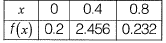By Simpson’s 1/3 Rule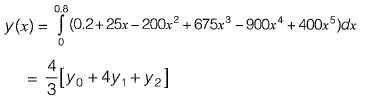0 = y(0) = 0.2
y1 = y (0.4) = 2.456
y2 = y(0.8) = 0.232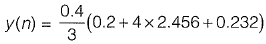= 1.367

Question 6: In Newton-Raphson iterative method, the initial guess value (xini) is considered as zero while finding the roots of the equation:
f(x) = -2 + 6x - 4X2 + 0.5.x3.
The correction, Δx, to be added to xini in the first iteration is ___________.
[2015 : 1 Mark, Set-II]

Solution:
f (x) = - 2 + 6x - 4x2 + 0.5x3
f '(x) = 6 - 8x + 1 .5x2
xini = 0
By Newton Raphson Method,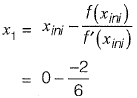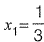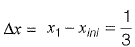Question 7: The quadratic equation x2 - 4x + 4 = 0 is to be solved numerically, starting with the initial guess x0 = 3. The Newton-Raphson method is applied once to get a new estimate and then the Secant method is applied once using the initial guess and this new estimate. The estimated value of the root after the application of the Secant method is  _____.    [2015 : 2 Marks, Set-I]
Solution.
f(x) = x2 - 4x + 4
f'(x) = 2x - 4
x0 = 3
f (3) = 1, f'(3) = 2
By Newton Rapshon method,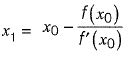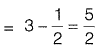f(5/2) = 25/4 -10 + 4 = 1/4
By Secant method,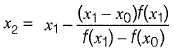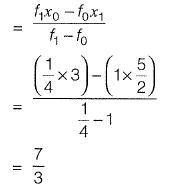Question 8: The integral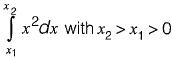is evaluated analytically as well as numerically using a single application of the trapezoidal rule. If I is the exact value of the integral obtained analytically and J is the approximate value obtained using the trapezoidal rule, which of the following statements is correct about their relationship?
(a) J > I
(b) J < I
(c) J = I
(d) insufficient data to determine the relationship
[2015 : 1 Mark, Set-I]
Answer: (a)
Solution: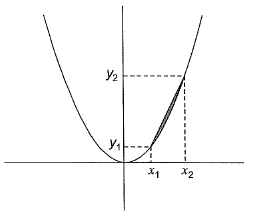Exact value is computed by integration which follows thee exact shape of graph while computing the area.
Whereas, in Trapezoidal rule, the lines joining each points are considered straight lines which is not the exact variation of graph all the time like as shown in figure.
∴ J > I
OR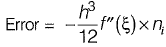Here, f(x) = x2
or,  f'(x) = 2x
or, f''(x) = 2 > 0
Since f''(x) is positive, the error is negative.
Since error = exact - approximate.
= I - J
and since error is negative in this case J > I is true.

Question 9: The magnitude as the error (correct to two decimal places) in the estimation of following integral using simpson 1/3 rule. Take the step length as 1.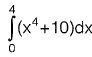[2013 : 2 Marks]
Solution: Using Simpson’s Rule,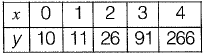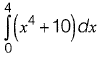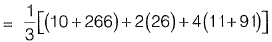= 245.33
The value of integral,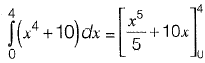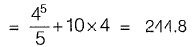∴Magnitude of error = 245.33 - 244.8 = 0.53

Question 10: The error in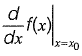for a continuous function estimated with h = 0.03 using the central difference formula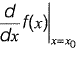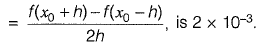The values of  x0 and f(x0) are 19.78 and 500.01, respectively. The corresponding error in the central difference estimate for h = 0.02 is approximately.
(a) 1.3 x 10-4
(b) 3.0 x 10-4
(c) 4.5 x 10-4
(d) 9.0 x 10-4
[2011 : 2 Marks]
Answer: (d)
Solution: Error in central difference formula is 0(h2)
This means,
error ∝ h2
If error for h = 0.03 is 2 x 10-3 then,
Error for h = 0.02 is approximately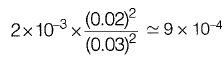Question 11: The estimate of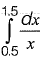Obtained using Simpson's rule with three-point function evaluation exceeds the exact value by
(a) 0.235
(b) 0.068
(c) 0.024
(d) 0.012
[2011 : 2 Marks]
Answer: (d)
Solution: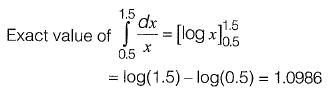Approximate value by Simpson’s rule with 3 point is,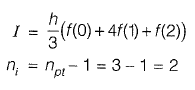(npt is the number of pts and ni is the number of intervals)
Hero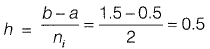The tabic is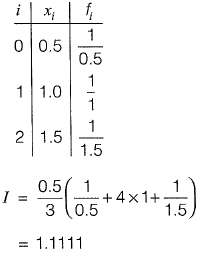So the estimate exceeds the exact value by,
Approximate value - Exact value
= 0.012499
≈ 0.012

Question 12: The square root of a number N is to be obtained by applying the Newton Raphson iterations to the equation x2 - N = 0. If i denotes the iteration index, the correct iterative scheme will be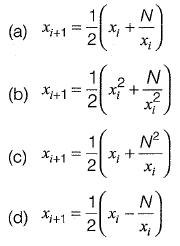[2011 : 1 Mark]
Answer: (a)
Solution: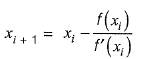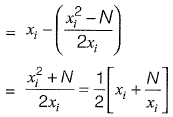Question 13: The table below gives values of a function F(x) obtained for values of x at intervals of 0.25.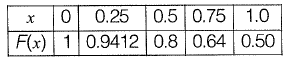The value of the integral of the function between the limits 0 to 1 using Simpson’s rule is
(a) 0.7854
(b) 2.3562
(c) 3.1416
(d) 7.5000
[2010 : 2 Marks]
Answer: (a)
Solution: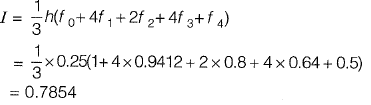Offer running on EduRev: Apply code STAYHOME200 to get INR 200 off on our premium plan EduRev Infinity!

69 docs

,

,

,

,

,

,

,

,

,

,

,

,

,

,

,

,

,

,

,

,

,

;